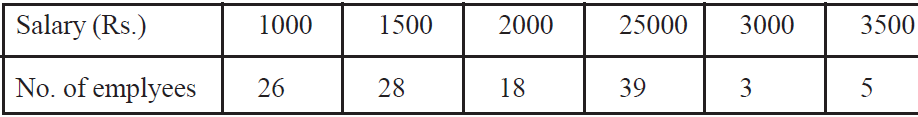# MP Board Class 11 Maths Important Questions

MP Class 11 Maths Important Questions help students to score high in the exams. These questions are gathered considering the important topics as per the MP Class 11 Maths Syllabus, and to update students knowledge about the subject.

Class 11 Maths Important Question also help students to understand the type of questions asked for the board exams. After proper analysis, we arrived at a conclusion that some of these questions are repeated often for the exams. It also helps students to get an approximate idea about the difficulty level of a paper.

MP Board Class 11 is a crucial year and Mathematics is an important subject for the students. The subject is difficult and will require a lot of practice, which is provided by these important questions. Students can start studying efficiently after taking a look at the important questions.

Students can also find a list of the important questions for MP board class 11 Maths here:

Important Questions for MP Class 11 Maths

1. Prove that
$$\begin{array}{l}i^{5}+i^{6}+i^{7}+i^{8}=0\end{array}$$
.
2. Calculate the value of a and b when:(i) (3,b)=(a,-1) (ii) (0,2)=(a-3, b+5)
3. Simplify
$$\begin{array}{l}\sqrt{4} \times (1-\sqrt{-64})\end{array}$$
.
4. Find the modulus of (4-3i)-(3+4i).
5. Express
$$\begin{array}{l}Cos 2\Theta\end{array}$$
and
$$\begin{array}{l}Sin 2\Theta\end{array}$$
in terms of
$$\begin{array}{l}Sin\Theta\end{array}$$
and
$$\begin{array}{l}Cos\Theta\end{array}$$
.
6. If roots of the equation
$$\begin{array}{l}ax^{2}+cx+c=0\end{array}$$
are in the ratio of p:q show that
$$\begin{array}{l}\sqrt{\frac{p}{q}}+\sqrt{\frac{q}{p}}+\sqrt{\frac{c}{a}}=0\end{array}$$
.
7. If the fifth and seventeenth term of an A.P. be 7 and 25 then find its 13th term.
8. Prove that the points A (a, b + c), B (b, c + a) and C (c, a + b) are collinear.
9. Write definition of non-singular and scalar matrix.
10. For a matrix A of order 2 × 2 A.(adj A) = then(a) 0 (b) 10 (c) 20 (d) 100
11. If A = then prove that AA ́ and A ́A are symmetric matrix, but AA ́ A´A.
12. OPQR is a square and M, N are the middle points of the sides PQ and QR respectively then the ratio of the areas of the square and triangle OMN is___________
13. The formula for Distance between two points is ?
14. Find the coordinate of incentre of triangle whose vertices are (2, –2), (8, –2) and (8, 6).
15. Find the equation of the straight line which has equal intercept on both the axis and form a triangle of area 8 sq. unit.
16. Find the distance between the straight lines y = 5x – 7 and y = 5x + 6
17. Write the equation of HYperbola.
18. Derive the equation of Parabola in standard form.
19. Prove that tan(A+30) + cot(A-30)=
20. Sketch the graph of y = sec 2x
21. Two places are due west of a leaning tower, which leans towards east are at a distance of “a” and “b” from its foot, if q and f are the elevations of the top of tower from these places. Prove that inclination to the horizontal is given bycot a =
22. For this distribution, find the mean, median and mode.23. How many straight lines can be obtained by joining 12 points out of which 5 points are collinear ? Also find that from these points now many triangles can be formed.
24. A man has two machines by which he can make either bottles or tumble to make bottles he has to run first machine for one minute and second for 2 minutes. To make tumblr he has to run each machine for one minute. Ist machine cannot be used for more than 50 minutes while other is for 54 minutes, he earns profit of 10 paise per bottle and 6 paise per tumblr. Assuming that he can sell all the items that he produces. Make mathematical model for number of items for the maximum benefit.

For more such resources and study material for MP Class 11, students can reach out to BYJU’S.